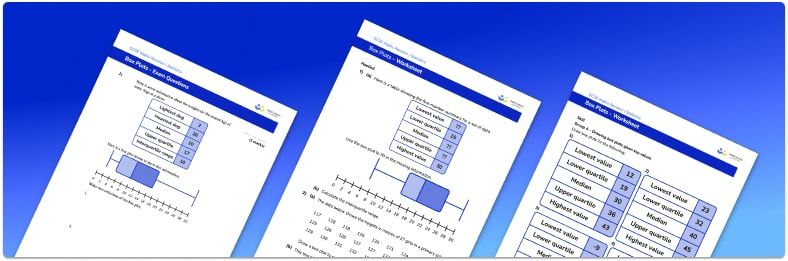# Box Plots Worksheet• Section 1 of the box plots worksheet contains 11 skills-based box plots questions, in 3 groups to support differentiation
• Section 2 contains 4 applied box plots questions with a mix of word problems and deeper problem solving questions
• Section 3 contains 3 foundation and higher level GCSE exam style box plots questions
• Answers and a mark scheme for all box plots questions are provided
• Questions follow variation theory with plenty of opportunities for students to work independently at their own level
• All questions created by fully qualified expert secondary maths teachers

Suitable for GCSE maths revision for AQA, OCR and Edexcel exam boards

• This field is for validation purposes and should be left unchanged.

You can unsubscribe at any time (each email we send will contain an easy way to unsubscribe). To find out more about how we use your data, see our privacy policy.

### Box plots at a glance

The five number summary set of statistics comprises the following values: the lowest value, the lower quartile, the median, the upper quartile and the highest value. These values could be decimals or integers, depending on the type of data being analysed. A box plot (or box and whisker plot) is a graphical representation of this summary. It is drawn on a grid with a horizontal scale marked along the bottom and allows us to view the distribution of the data.

The vertical edges of the box are the lower quartile and the upper quartile. The median is marked with a vertical line in between these two values. The box is completed with horizontal lines. The whiskers run to the lower extreme (minimum value) and upper extreme (maximum value) of the data set. At GCSE, students are not expected to identify or mark outliers, but these data points can sometimes be seen as crosses marked outside the range of the whiskers.

Along with constructing box plots from given data, a key skill is interpreting box plots and discussing the distribution shown. When comparing sets of data, it is useful to compare medians and the IQR (interquartile range) of the data sets.

The lower quartile, or first quartile, is 25% (one quarter) of the way through the data, and the upper quartile, or third quartile, is 75% (three quarters) of the way through the data, exactly half of the data lies between the first quartile and third quartile

If a set of data has a large interquartile range, the middle 50% of data is more spread out. Conversely, if the set of data has a small interquartile range, the middle 50% of the data values are closer together.

Looking forward, students can then progress to additional Statistics worksheets, for example the averages from frequency tables worksheet, the mean from a frequency table worksheet, or the quartiles worksheet.

For more teaching and learning support on Statistics our GCSE maths lessons provide step by step support for all GCSE maths concepts.

## Do you have KS4 students who need more focused attention to succeed at GCSE?There will be students in your class who require individual attention to help them succeed in their maths GCSEs. In a class of 30, it’s not always easy to provide.

Help your students feel confident with exam-style questions and the strategies they’ll need to answer them correctly with our dedicated GCSE maths revision programme.

Lessons are selected to provide support where each student needs it most, and specially-trained GCSE maths tutors adapt the pitch and pace of each lesson. This ensures a personalised revision programme that raises grades and boosts confidence.

Find out more